# Precalculus : Find the Degree Measure of an Angle

## Example Questions

### Example Question #71 : Trigonometric Functions

Convert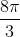radians to degrees.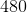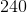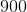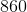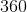Explanation:

Write the conversion factor between radians and degrees.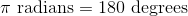Cancel the radians unit by using dimensional analysis.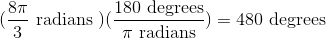### Example Question #1 : Angles In The Coordinate Plane

Convert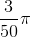to degrees.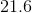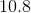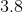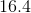Explanation:

Write the conversion factor of radians and degrees.Substitute the degree measure into.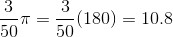### Example Question #1 : Find The Degree Measure Of An Angle

Determine the angle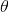in degres made in the plane by connecting a line segment from the origin to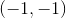.

Assume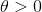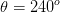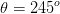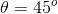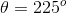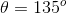Explanation:

Firstly, since the pointis in the 3rd quadrant, it'll be between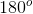and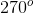. If we taketo be the horizontal, we can form a triangle with base and leg of valuesand. Solving for the angle in the 3rd quadrant given by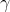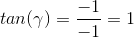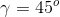Since this angle is made by assumingto be the horizontal, the total angle measureis going to be: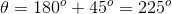### Example Question #2 : Find The Degree Measure Of An Angle

Find the degree measure of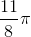radians.  Round to the nearest integer.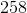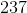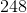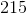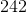Explanation:

In order to solve for the degree measure from radians, replace theradians with 180 degrees.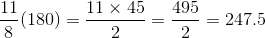The nearest degree is.

### Example Question #3 : Find The Degree Measure Of An Angle

Given a triangle, the first angle is three times the value of the second angle.  The third angle is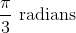.  What is the value of the second largest angle in degrees?Explanation:

A triangle has three angles that will add up todegrees.

Convert the radians angle to degrees by substituting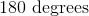for every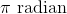.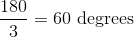The third angle is 60 degrees.

Let the second angle be.  The first angle three times the value of the second angle is.  Set up an equation that sums the three angles to.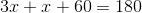Solve for.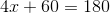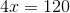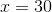Substitutefor the first angle and second angle.

The second angle is:The first angle is: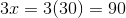The three angles are: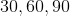The second highest angle is:### All Precalculus Resources